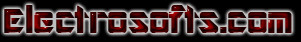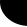HOME Electronics Directory Articles/ Tutorials eBooks About Us FORUM Links Contact UsFuzzy Logic Tutorial Part I:                                                 INTRODUCTION TO FUZZY LOGIC

The idea of fuzzy logic was born in 1965. Lofti A. Zadeh published a seminar paper on fuzzy sets which was the birth of fuzzy logic technology. At the beginning fuzzy logic was strongly resisted, but step by step more studies had been performed. In the decade after Dr. Zadeth’s seminal paper many theoretical developments in fuzzy logic took place in the United States, Europe and Japan. These exploited the fact that for example Fuzzy Logic is tolerant of imprecise data, can model nonlinear functions and can be blended with conventional control techniques.

Fuzzy Logic is a technique that allows us to map an input space to an output space, Similar to a black box which does ‘something’ to compute the solution, the output values. As shown in Figure 1 below:Figure 1: Black box model: Input-Output System.

There are many ways in which we can implement the black box: neural networks, linear systems, expert systems, differential equations, and so on.

So, why should we use Fuzzy Logic? The basic answer is because basically it is more understandable, faster and cheaper than other technologies.

The main principle of fuzzy logic relates to the use of fuzzy sets, which are classes with (unsharp) no crisp boundaries. For instance, to define the set of tall people, we could proceed as shown in Figure 2 below.Figure 2: Set of tall people.

A sharp boundary would consider a ‘tall person’ to be taller than, for instance, 170cm. And it would consider a ‘short person’ to be shorter than 170cm. Therefore if we have two people, one 169.8cm of tall and the other one 170.1cm of tall, we would consider the first one to be a short person and the second one to be a tall person. It has no sense to consider one person tall and another short if the difference between them is only 0.3cm.

As regards Figure 2, using a crisp or sharp boundary we would consider person 2 tall and all the other people short.

The human reasoning doesn’t work in that way. We don’t say that the person number2 is tall and the number 3 isn’t, because it doesn’t seem sensible. Both number 2 and 3 have, more or less, the same height so we can’t consider one tall and the other one short. We have degrees between tall and short. Likewise we can’t consider the number 1 short and the number four also short, because we consider number four not really tall, but in no way as short as number 1.

Human reasoning has “degrees” between different concepts; we don’t always have crisp boundaries. Fuzzy logic allows us to work like we think, so it’s conceptually easy to understand. The mathematical concepts behind fuzzy reasoning are very simple, which makes Fuzzy Logic very natural. It is based on both on natural reasoning and on natural language.

Fuzzy logic generalizes the binary distribution between possible and impossible to a matter of degree called the possibility.

Nowadays Fuzzy Logic affects many disciplines: videography (Sanyo), air-conditioning (Mitsubishi), and washing-machines (combining smart sensors with Fuzzy Logic by Matsushita), for cars Nissan introduced a fuzzy automatic transmission. There are also fuzzy toasters, fuzzy rice-cookers, and so on.

There are applications of fuzzy system theory to telecommunications such as channel equalization or call acceptance, signal processing such as fuzzy filters or fuzzy signal detection, fuzzy systems in control engineering, such as well working together with neural networks. As we can see Fuzzy Logic has become widely used and this shows its usefulness.

Section 2 is about linguistic variables, fuzzy membership functions, that is the basic elements that allow us to build Fuzzy Logic Systems.

(You can contact author through email: vadiraj@electrosofts.com )Web electroSofts.com
 Home   |    About Us   |   Articles/ Tutorials   |   Downloads   |   Feedback   |   Links   |   eBooks   |   Privacy Policy Copyright © 2005-2007 electroSofts.com. webmaster@electroSofts.com